# How to: handle capacity constraints for different commodities measured in different units

I have been working on a vehicle routing problem in which the truck transports several commodities, but these are measured in different units. Hence, we know the capacity of the truck for each of these commodities alone, but we don’t know how to convert them into one another so that we can load two commodities and still respect the capacity.

For the sake of this explanation, consider the following example. We have a basket that can hold 2 watermelons or 10 oranges. Letbe the number of watermelons in the basket,be the number of oranges, and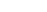and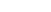be the respective capacities.

It is easy to observe that 1 watermelon is equivalent to 5 oranges, and if there is 1 watermelon in the basket, you could still add up to 5 oranges. In math terms, that is: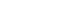or yet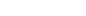.

Generalizing this idea, let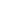and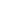be the number and the capacity of commodity. The combined capacity constraint becomes: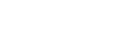for a given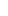.

Observe how the LHS converts all use of the resource intoterms, and the RHS considers the capacity for commodity.

Note that the LHS will likely contain fractional numbers, which can cause numerical instabilities when solving the resulting MIP. This will definitely happen if you simplify the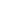terms in the LHS and RHS. However, to decrease this probability and if the capacities are multiples of a given number, you can avoid these fractions by using the largest: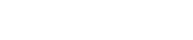.

Thanks Gafa!

This was useful? Buy me a cup of coffee to help me keep this website running!